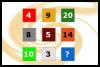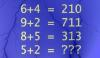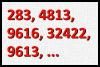BRAIN TEASERSBrain Teasers User Profile

# Amit Jain

rank
425
points
5
See full ranking list
short ranking list
 423 CU29 Uroš 5 424 Fernando Salazar 5 425 Amit Jain 5 426 tayyab Amjad 3B 5 427 Wheelie Boy 5
 Which number should replace the question mark? Which number should replace the question mark?Mathematical Puzzle: IF 6+4=... Mathematical Puzzle: IF 6+4=210, 9+2=711 and 8+5=313 THEN 5+2=?What is the next number in the sequence? What is the next number in the sequence (283, 4813, 9616, 32422, 9613, ...)?